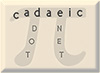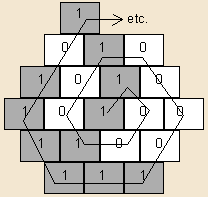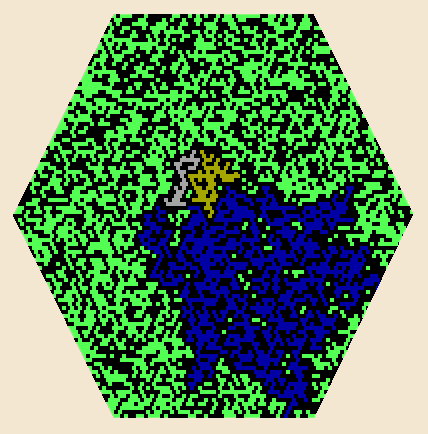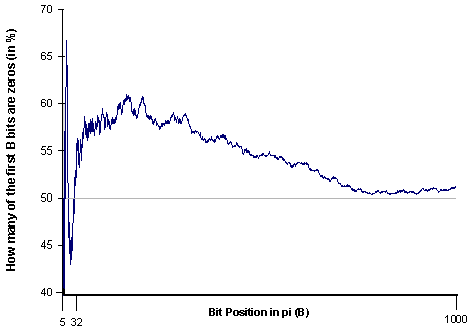Lady Pi Mike Keith June 1999

Sure, the digits of the number pi (3.14159265358979...) are a random-looking primordial soup. But did you know there's a picture of a person hiding in there? Here's how to uncover it.

This discovery was first made in 1998 by Richard Lawrence of Schuyler Falls, New York, working entirely by hand. He took the value of pi expressed in binary, which begins

11.001001000011111101101010100010001000010110100011000010001101001100010011...

and arranged the binary digits in order on a spiral within a hexagonal lattice, like so:Figure 1. Binary pi on a hexagonal spiral.

Then, he colored in the locations of the "1" bits, as shown in the figure. After extending the plot to about 15 revolutions of the spiral, he noticed what appeared to be a "face" just above the center of the plot. The face has two eyes, an open mouth, and even appears to have hair flowing off to the right. What happens, we wondered, if the plot is continued?

The result is shown below.Figure 2. Lady pi walks in the Sunlit Garden.

After drawing 50 revolutions of the binary-pi spiral, we have colored in three areas using a flood fill. Voila! - it's Lady Pi. She has a face with two eyes (and, well, yes, kind of an odd-shaped mouth), hair streaming off to her left, a long flowing robe, and - most dramatic - her right hand is lifted up to sheild her eyes from the sun. The center of the spiral shown in Figure 1 is right at the base of her "neck".

Lest you think we cheated, here is the entire code to draw Figure 2, written in UBASIC, a public-domain version of BASIC that handles high-precision numbers (and has the value of pi built in). The program converts pi to binary (see line 160, which computes the next bit in the sequence), plots the spiral, and does the coloring. Try it yourself! (After it draws, hit a key to return to text mode.)

2 ' Program to plot binary pi spiral and reveal "Lady Pi"
4 ' Idea by Richard Lawrence; program by Mike Keith
5 '
6 point 530
7 Col=10
10 screen 23:cls 3
20 dim Dx(6),Dy(6)
30 Dx(0)=2:Dx(1)=1:Dx(2)=-1:Dx(3)=-2:Dx(4)=-1:Dx(5)=1
40 Dy(0)=0:Dy(1)=2:Dy(2)=2:Dy(3)=0:Dy(4)=-2:Dy(5)=-2
70 X=320:Y=240 ' center point
90 Pival=#pi-2
100 for Shell=1 to 50
110 X=X+Dx(4):Y=Y+Dy(4)
120 for K=0 to 5
130 for N=1 to Shell
140 X=X+Dx(K):Y=Y+Dy(K)
150 if (int(Pival)=0) line (X,Y)-(X+1,Y+1),Col,"bf",Col
160 Pival=(Pival-int(Pival))*2
170 next N
180 next K
190 next Shell
195 line (331,218)-(332,218),0,"bf",0
196 line (305,210)-(306,210),0,"bf",0
200 paint (320,224),6,0
210 paint (322,240),1,0
220 paint (305,212),7,0
225 paint (293,240),1,0
230 A=input\$(-1): console

Of course, the "discovery" of this image just a combination of coincidence and our human ability to see patterns. But there is another feature of the binary digits of pi that's partly to blame for the image's clarity. Note the relative solidity of Lady Pi's head and hand, which are seemingly more solid than we would expect in a typical 50% mixture of black and color pixels. This is the result of a surprising statistical feature of the initial bits of pi. The number pi is conjectured by mathematicians to be "normal", which means that in the limit its digits, in any base, behave statistically like uniform random numbers. Thus, in the long run, we expect 50% zeros and 50% ones. However, the graph below shows the unexpected thing that happens near the beginning of pi.Figure 3. Zeros vs. ones in binary pi.

The vertical axis shows the percentage of zeros in the first B bits. After some initial wild swings, ending with a value of 50% at bit 32, the graph suddenly zooms up to a phenomenal peak of 61% at bit 164 and stays well above 50% for several hundred bits. It is still noticeably above 50% at bit 1000, and does not hit a value of exactly 50% again until bit number 6356 (about the number of bits in the Lady Pi picture). Since the 0 bits are the ones we color, there really are more large blobs of color in the picture than one would expect by chance. Add to that a dash of human imagination, and the end result is Lady Pi.